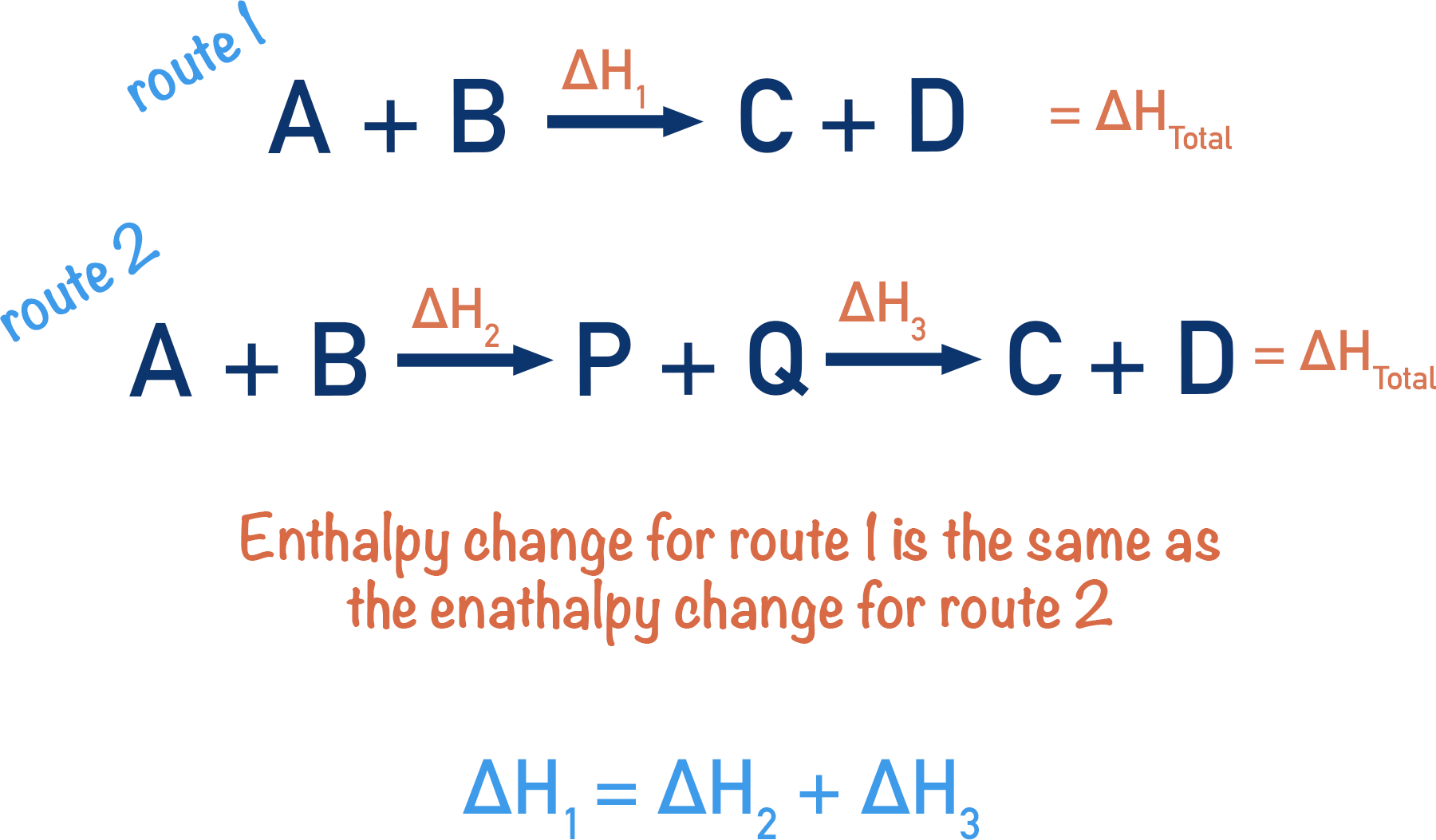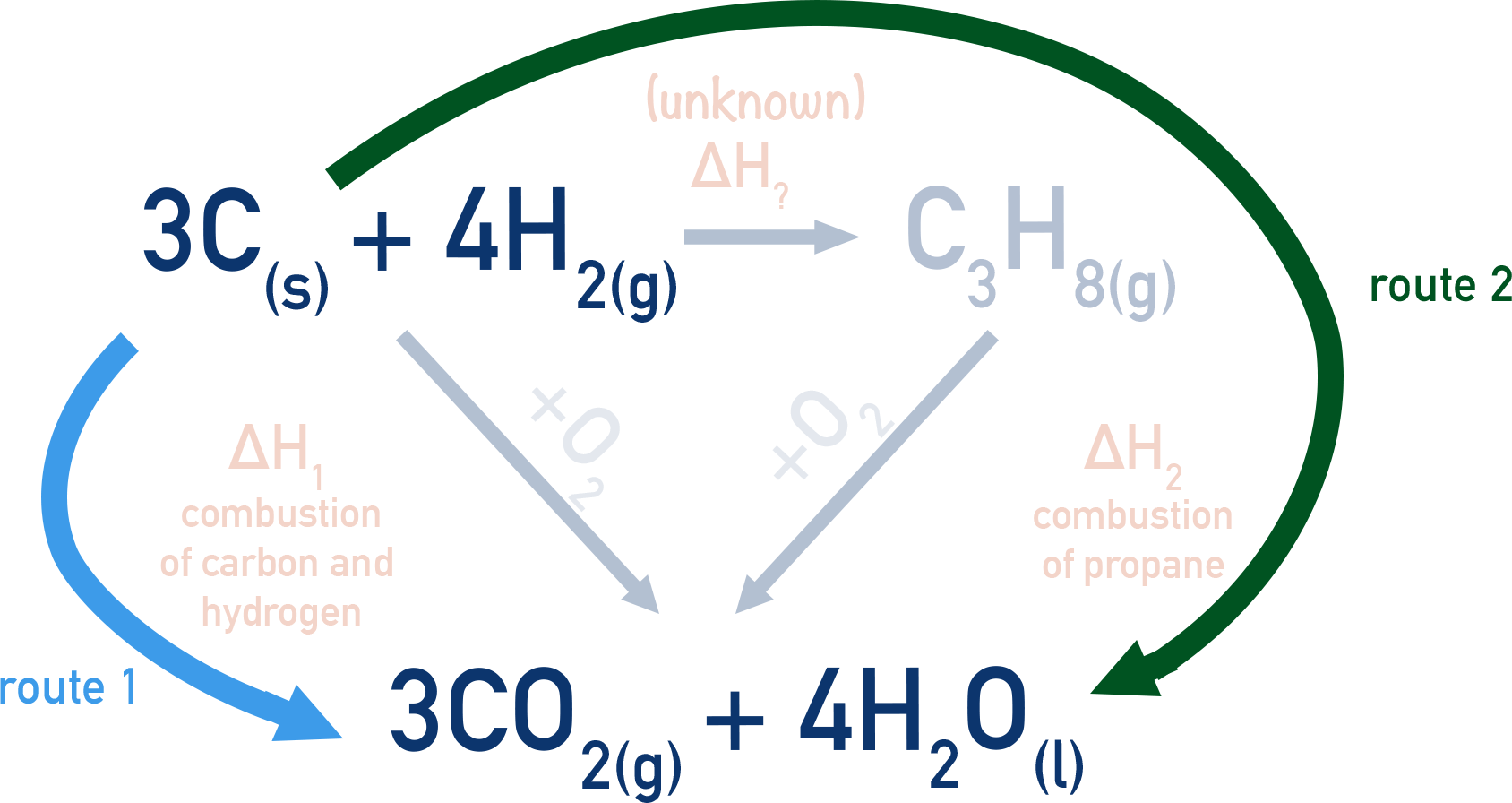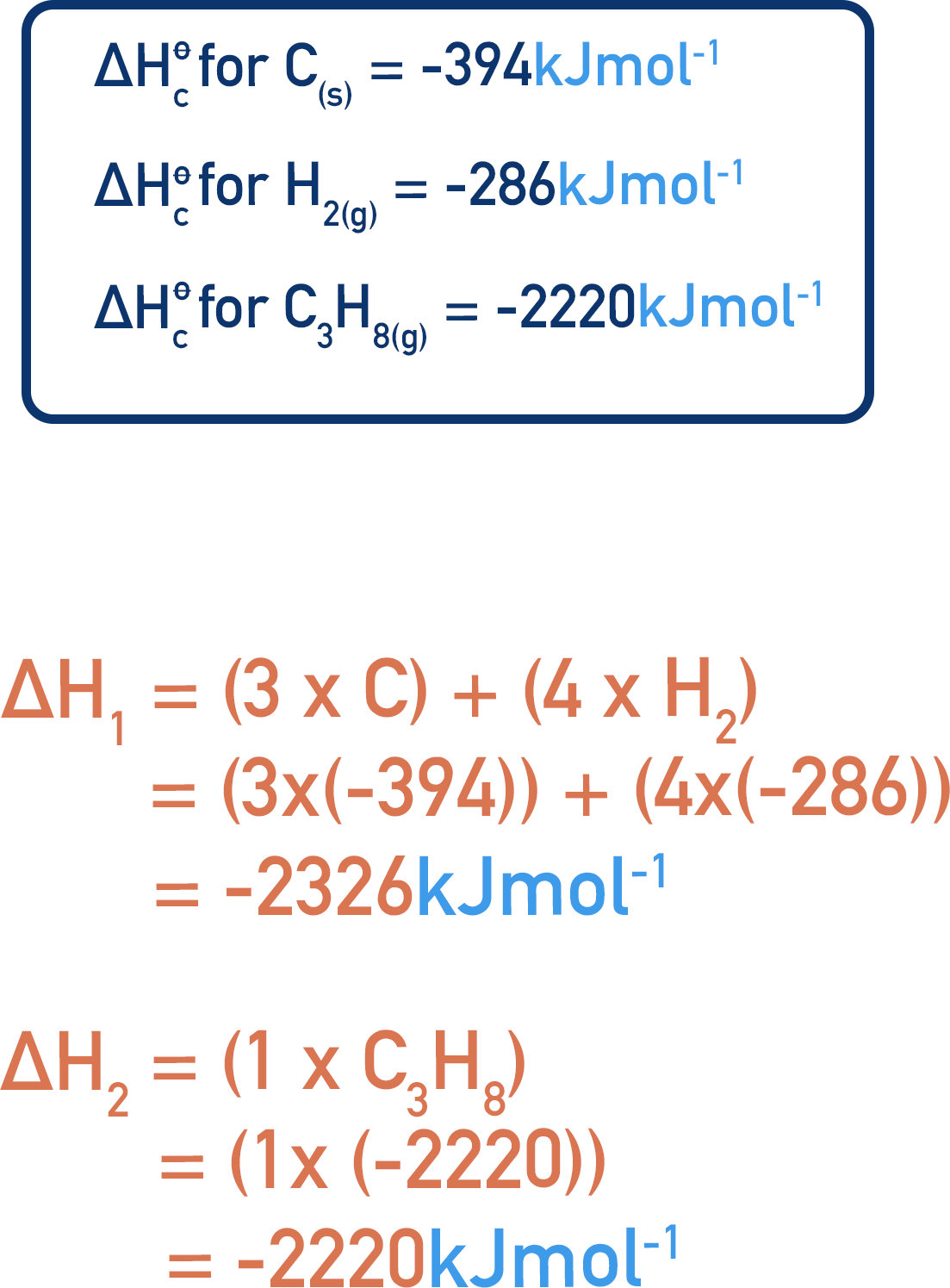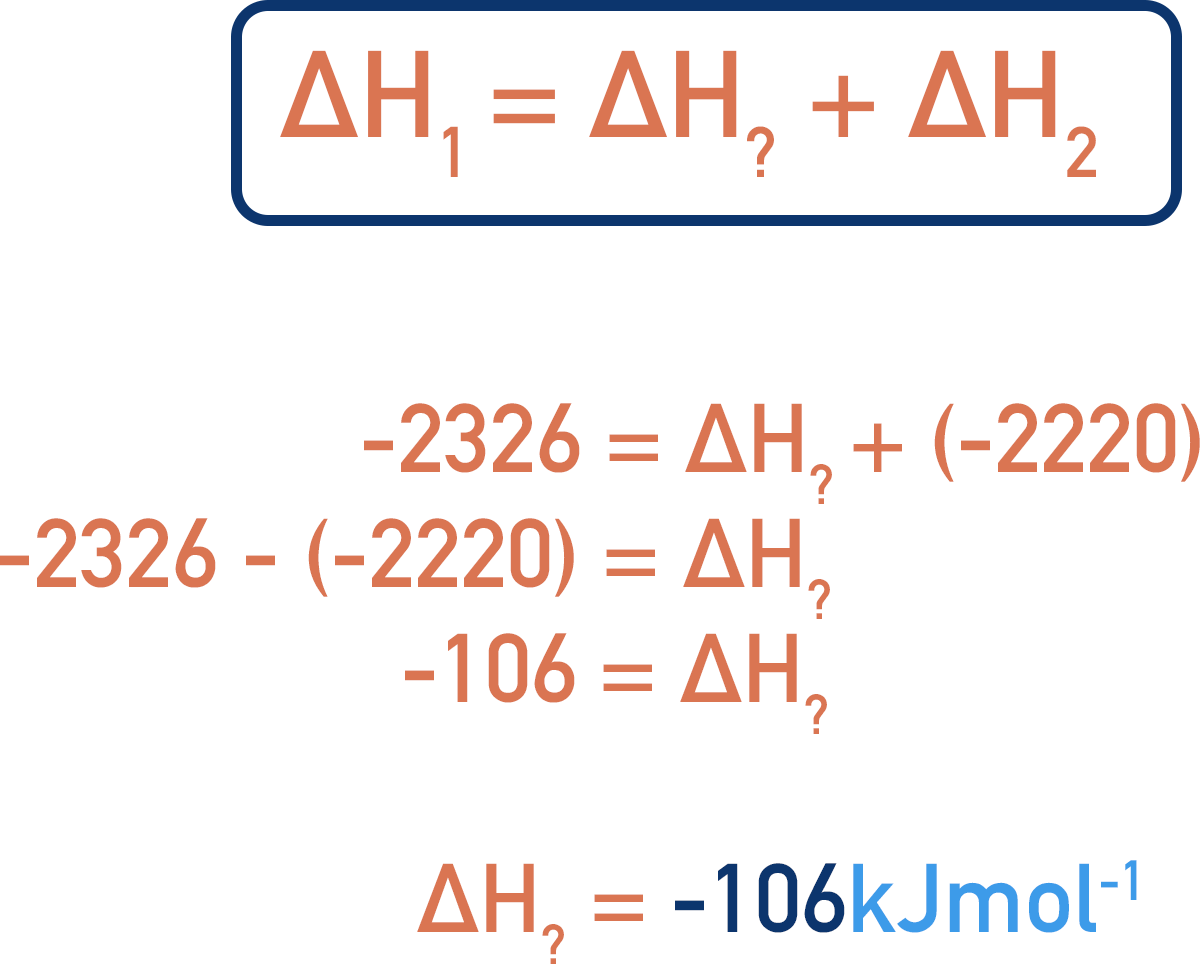Quick Notes Hess' Law

• Hess’ Law states that the energy change that occurs when a substance is changed into a product is the same, regardless of the route taken.
• There is often more than one way to go from a reactant to a product, meaning there is more than one ‘route’ to convert a substance to a particular product.
• Hess cycles are ways of drawing out the energy changes that occur when a substance is turned into a different substance via more than one route.

Full Notes Hess' Law

Very often in chemistry there is more than one route (way) to turn a substance into a product.

When a substance reacts to form a new product, a given amount of energy is either released or absorbed during the reaction.

This amount of energy is the same regardless of how we get to our end product. We call this Hess’ Law.

Hess’ Law - The enthalpy change for a reaction is always the same, regardless of the route taken.

Imagine having to get to the top of a mountain, you can either try to go straight up the mountain or walk around a path that winds up the mountain. With either route, the height you climbed would be exactly the same!

Now, imagine you ‘stop’ halfway up the mountain before using a different path again to get to the top. The height you climbed would still be the same as if you had gone straight up in one go. This is the same for enthalpy changes in reactions; it doesn’t matter HOW you get from a reactant to a product, the total enthalpy change will always be the same.If we know all the enthalpy changes for individual stages within a reaction process, we can calculate the overall enthalpy change. We can also rearrange the enthalpy changes to calculate enthalpy changes for a specific stage.

## Hess Cycles

By drawing out two potential routes to a product from reactants, we can find the enthalpy change for one of the routes if the other enthalpy change is known.

For example, the formation of propane (C3H8) from hydrogen (H2) and carbon (C) can be found by using a Hess cycle and combustion data.To make one mole of propane (C3H8), three moles of carbon (C) and four moles of hydrogen (H2) are needed.

We can measure the enthalpy change that occurs when one mole of propane is combusted (reacted fully in oxygen), and we can measure the combined enthalpy change that occurs when three moles of carbon and four moles of hydrogen are combusted.

### Standard enthalpy changes

When one mole of a substance is combusted fully with oxygen, under standard conditions (298K and 101KPa), the enthalpy change measured is referred to as a ‘standard enthalpy of combustion’ and is given the symbol (ΔHc). The ⦵ symbol is used to represent standard conditions and the C shows it is a combustion reaction. Standard enthalpies are very useful because they mean we can use data-book values rather than actually combusting substances ourselves to solve a Hess cycle.

There are many standard enthalpy changes in chemistry, the two you usually need to know for Hess cycles are standard enthalpies of combustion and standard enthalpies of formation. For more advanced standard enthalpies, see Born-Haber cycles.

Standard enthalpy of combustion = enthalpy change that occurs when one mole of a substance reacts completely with oxygen at standard conditions.

Standard enthalpy of formation = enthalpy change that occurs when one mole of a substance is formed from its constituent elements at standard conditions.

By forming a cycle (see diagram), we can use enthalpies of combustion data to find the enthalpy change of the formation of propane.

Remember: Hess’ law states that the enthalpy change of a reaction is the same, regardless of which route we take to form the products.

In this case, we are producing carbon dioxide and water from carbon and hydrogen by two different routes. The first route is to convert three moles of carbon and four moles of hydrogen into propane, then combust the propane. The second route is to combust three moles of carbon and four moles of hydrogen directly.Hess’s law tells us the overall enthalpy change for both routes will be the same.If we know how much energy change occurs when we combust carbon, hydrogen and propane, we can calculate the enthalpy changes for our known steps.Now, it’s just a question of using our original cycle to re-arrange the equation shown to find our unknown enthalpy change for the production of propane from carbon and hydrogen.